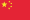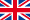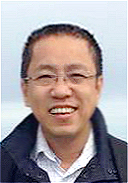中文English## Professors

 Jianhai Bao William Y.C. Chen Yuping Duan Zaihui Gan Kathy Q. Ji Renjin Jiang Kangwei Li Jinghai Shao Xiaotao Sun Fengyu Wang Gengsheng Wang Ou Wu Yifei Wu Yong Zhang Chuanming Zong

 Hua Chen Peter Paule

## Associate Professors

 Song Dai Xiequan Fan Ling He Eryan Hu Huaiqian Li Zhenling Peng Ruipeng Shen Baofang Song WenYi Tian Yaohong Wang Huaming Wu Tian Xu Xun Yu Haixiang Zhang

## Assistant Professors

 Xin Chen Yingjun Deng Alan J.X. Guo Haoyang Huang Xing Huang Cong Liang Wenjun Ma Bin Wei Jie Wu Manting Xie Song Yang Yubiao Zhang Mingfeng Zhao Mingshuo Zhou Fei Zhu

## Post-Doctors

 Lingyan Cheng Teng Fang Chao Ji Hongying Lin Jijian Song Dazhao Tang Lixia Wang Jiakuan Xu Xu Zhang

 Albert Y. Liu Boran Li Xiaoping Zhong### Email: wanggs62@yeah.net; wanggs@tju.edu.cn

Research Interests
• Control theory in distributed systems, in particular, time optimal controls, periodic feedback stabilization and controllability. Recently, more concerns on sampled-data controls and impulsive controls

• Heat kernel estimates

• Eigenvalue estimates

Education
• 1979.09―1983.06 B.S., Mathematics, Wuhan University, China

• 1983.09―1986.06 M.Sc., Mathematics, Wuhan University, China

• 1989.09―1994.06 Ph.D, Applied Mathematics, Ohio University, USA
Editorships and Reviewing Activities
• Associate Editor, SIAM J. Control and Optimization, 2018.12.31-2020.12.31.

• Associate Editor, ESIAM, Control, Optimization and Calculus, 2009-Present.

• Associate Editor, Mathematical Control and Related Fields. 2011-present.
Publications

(a) Monographs
• G. Wang, L. Wang, Y. Xu and Y. Zhang, Time Optimal Control of Evolution Equations. Progress in Nonlinear Differential Equations and Their Applications, 92. Subseries in Control, Birkh"{a}user/Springer, 2018.

• G. Wang and Y. Xu, Periodic Feedback Stabilization for Linear Periodic Evolution Equations, SpringerBriefs in Mathematics,ISBN 978-3-319-49237-7, DOI 10.1007/978-3-319-49238-4, 2016.
(b) Papers
• G. Wang, M. Wang, C. Zhang and Y. Zhang, Observable set, observability, interpolation inequality and spectral inequality for the heat equation in $mathbb{R}^n$, Journal de Mathématiques Pures et Appliquées, to appear.

• G. Wang, M. Wang and Y. Zhang, Observability and unique continuation inequalitiesfor the Schrodinger equation, J. Eur. Math. Soc., to appear.

• S. Qing and G. Wang,Equivalence between minimal time and minimal norm control problems for the heat equation, SIAM J. Control and Optim.,56 (2018) 981-1018.

• G. Wang, D. Yang and Y. Zhang, Time optimal sampled-data controls for the heat equation, C. R. Acad. Sci. Paris. Ser. I 355 (2017) 1252-1290.

• S. Qing and G. Wang, Controllability of impulse controlled systems of heat equations coupled by constant matrices, J. Differential Equations, 263 (2017) 6456-6495.

• K. D. Wang, G. Wang and Y. Xu, Impulse output rapid stabilization for heat equations, J. Differential Equations, 263 (2017) 5012-5041.

• G. Wang and C. Zhang, Observability inequalities from measurable sets for some abstract evolution equations. SIAM J. Control and Optim. 55 (2017) 1862-1886.

• G. Wang and Y. Zhang, DECOMPOSITIONS AND BANG-BANG PROPERTIES, MathematicalControl and Related Field, Vol 7. No. 1 (2017) 73-170.

• M. Tucsnak, G. Wang and C. Wu, Perturbations of time optimal control problems for a class of abstract parabolic systems, SIAM J. Control and Optim., 54 (2016) 2965-2991.

• G. Wang, Y. Xu and Y. Zhang, Attainable subspaces and the bang-bang property of time optimal controls for heat equations, SIAM J. Control and Optim., 53 (2015) 592-621.

• W. Gong, G. Wang and N. Yan, Approximations of elliptic optimal control problems with controls acting on a lower dimensional manifold, SIAM J. Control and Optim., 52 (2014) 97-119.

• G. Wang and Y. Xu, Equivalent conditions on periodic feedback stabilization for linear periodic evolution equations, J. Funct. Anal., 266 (2014) 5126-5173.

• J. Apraiz, L. Escauriaza, G.Wang and C. Zhang, Observability inequalities and measurable sets, J. Eur. Math. Soc., 16 (2014) 2433-2475.

• G. Wang and Y. Xu, Periodic stabilization for linear time-periodic ordinary differential equations, ESAIM COCV, 20 (2014) 269-314.

• P. Lin and G. Wang, Properties for some blowup parabolic equations and their applications. Journal de Mathématiques Pures et Appliquées, 101(2014) 223-255.

• K-D Phung and G. Wang, An observability estimate for parabolic equations from a measurable set in time and its applications, J. Eur. Math. Soc., 15,2 (2013) 681-703.

• G. Wang and Y. Xu, Equivalence of three different kinds of optimal control problems and its applications, SIAM J. Control and Optim., 51 (2013) 848-880.

• G. Wang and E. Zuazua, On the equivalence of minimal time and minimal norm controls for internally controlled heat equations, SIAM J. Control and Optim., 50 (2012) 2938-2958.

• G. Wang and G. Zheng, An approach to the optimal time for a time optimal control problem of an internally controlled heat equation. SIAM J. Control and Optim., 50 (2012) 601-628.

• Q. Lv and G. Wang, On the existence of time optimal controls with constraints of the rectangular type for heat equations. SIAM J. Control and Optim., 49 (2011) 1124-1149.

• P. Lin and G. Wang, Blowup time optimal control for ordinary differential equations. SIAM J. Control and Optim., 49 (2011) 73-105.

• K-D Phung and G. Wang, Quantitative unique continuation for the semilinear heat equation in a convex domain, J. Funct. Anal.，259 (2010) 1230-1247.

• G. Wang, $L^infty$-null controllability for the heat equation and its consequences for the time optimal control. SIAM J. Control and Optim., 47 (2008) 1701-1720.

• G. Wang and D. Yang, Decomposition of vector-valued divergence free Sobolev functions and shape optimization for stationary Navier-Stokes equations. Comm. PDE, 33 (2008) 1-21.

• L. Lei and G.S. Wang, Optimal control of semilinear parabolic equations with k-approximate periodic solutions. SIAM J. Control and Optim., 46 (2007) 1754-1778.

• K-D Phung, G. S. Wang and X.Zhang, Existence of time optimal control of evolution equations. Discrete and Continuous Dynamical Systems, Ser. B, Vol. 8, No. 4 (2007) 925-941.

• G. Wang, L. Wang and D. Yang, Shape optimization of elliptic equations in exterior domains. SIAM, J. Control and Optim., 45 (2006) 532-547.

• V. Barbu and G.S.Wang, Feedback stabilization of periodic solutions to nonlinear parabolic evolution systems.Indiana Uni. Math. J., 54, 6 (2005) 1521-1546.

• L. Wang and G. Wang, Time optimal control of Phase-field systems. SIAM J. Control And Optim., 42 (2003) 1483-1508.

• G. Wang, Optimal controls of 3-dimensional Navier-Stokes equations with state constraints. SIAM. J.Control and Optim., 41 (2002) 583-606.

• G. Wang and L. Wang, State-constrained optimal control governed by non-well posed semilinear parabolic differential equation. SIAM J. Control and Optimization, 40 (2002) 1517-1539.

• G. Wang, Optimal control of parabolic differential equations with two point boundary state constraints. SIAM J. Control Optim., 38 (2000) 1639-1654.

Center for Applied Mathematics, Tianjin University, No.92 Weijin Road, Tianjin 300072, P. R. China